# LM1B 9.2.6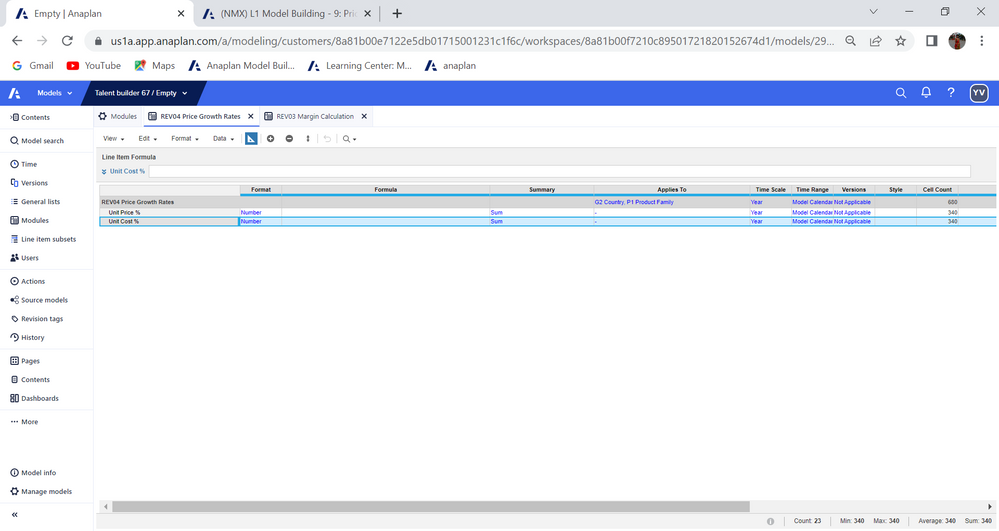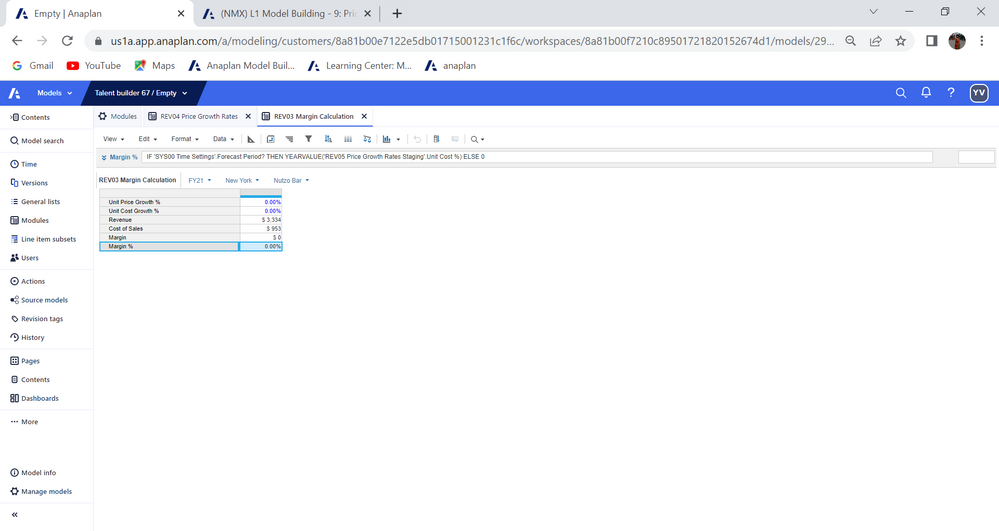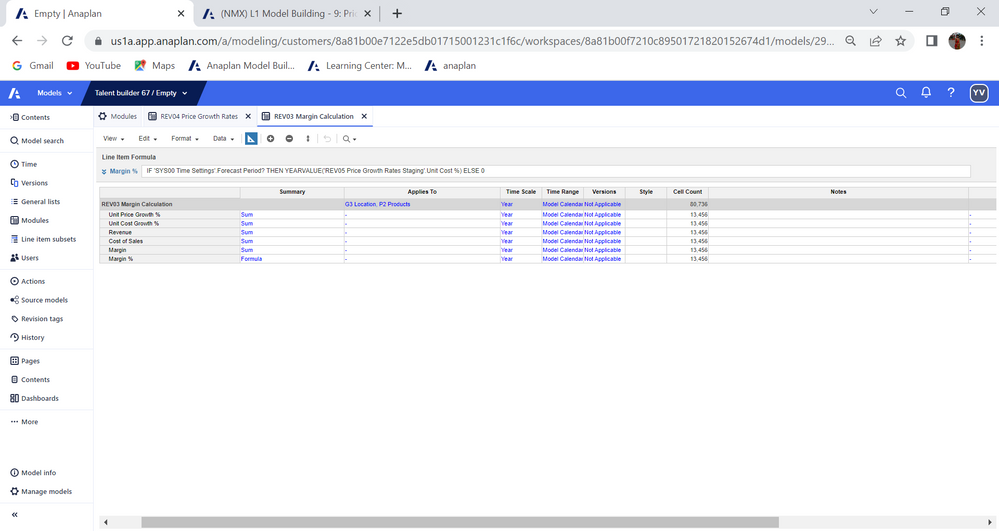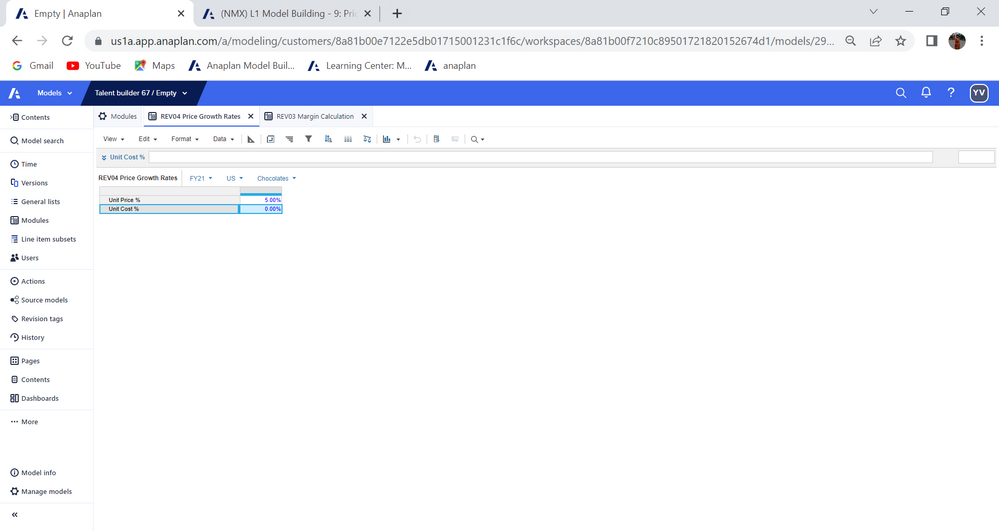carryforward problem

•Formula is correct. What error are you getting?

•5.00% is not getting carryforwarded
•Expected: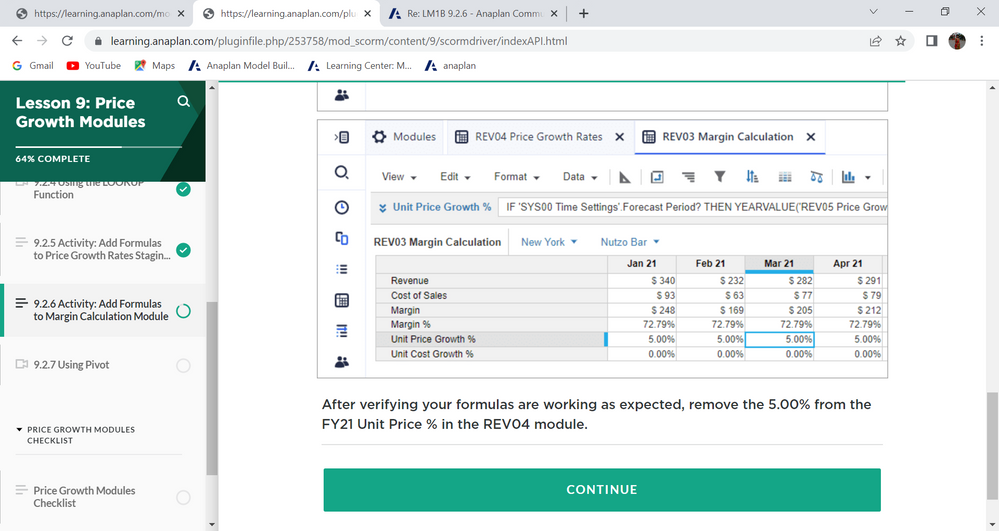this screenshot is part of activity, where unit price growth % has a formula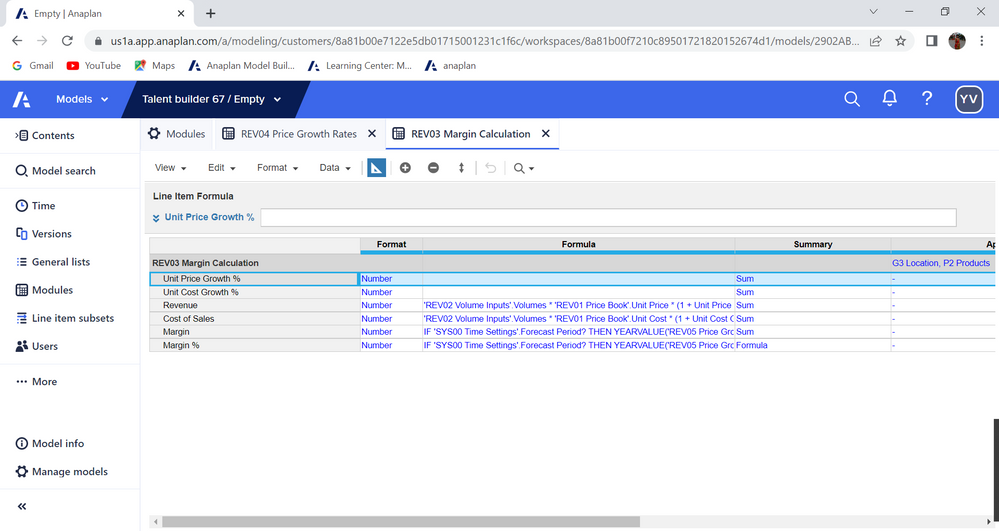Here, there is no formula . So shd i add a formula? formula from REV04 did not get carryforwarded to rev03

•Hi

You have put your IF formulas in the wrong line items

They belong in the unit cost and unit price % lines

You also will need to correct and use the right formulas for margin and margin %

Please refer to the activity again for guidance

Thank you

Ravinder

•can u please check, I changed the formulas

the value 5.00% is not getting carryforwarded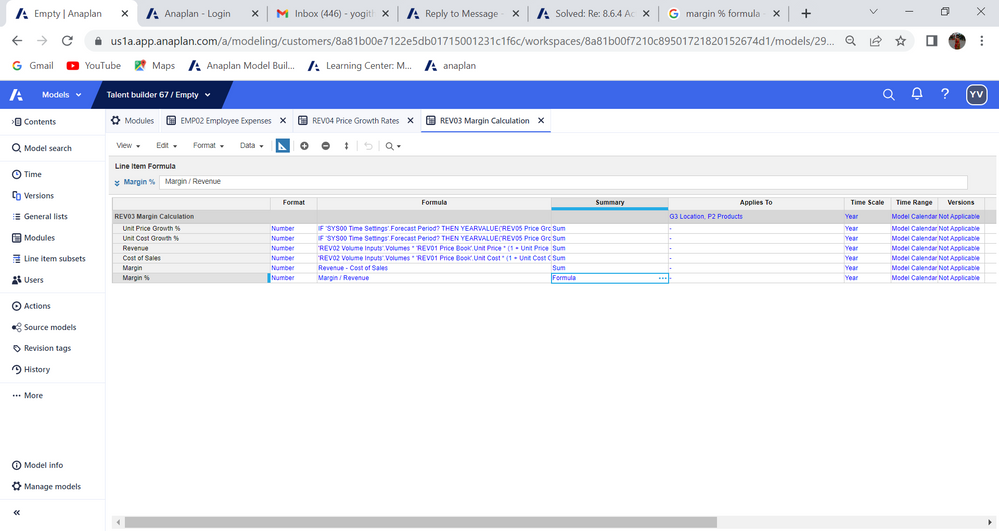•What are the 2 formulas in full for unit price growth % and unit cost growth %?

Remember you should input a % in price growth module for a detail value eg US and chocolates, and see the 5% in the price growth staging module in the related values, and see the 5% in the margin calc module; only in yr 20 or 21

Thank you

Ravinder

•Here are the for formulas:

I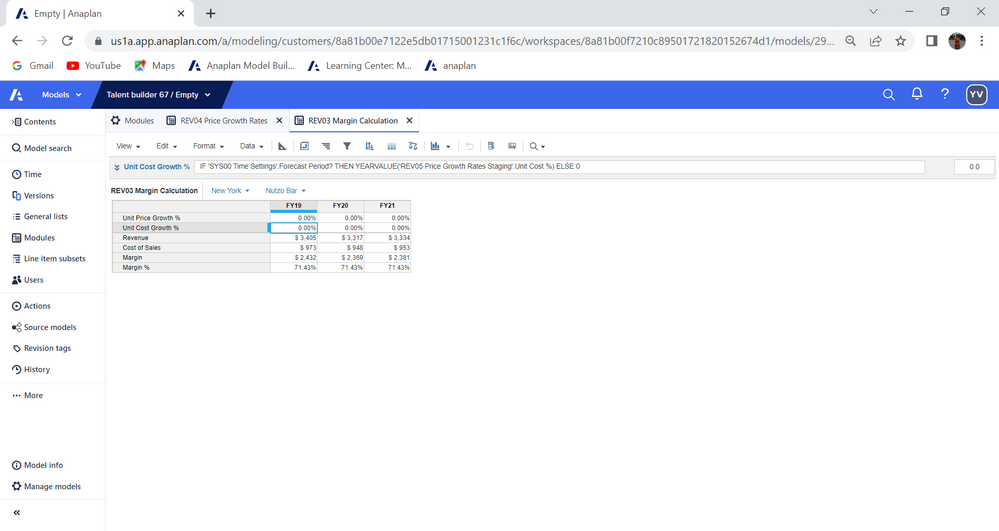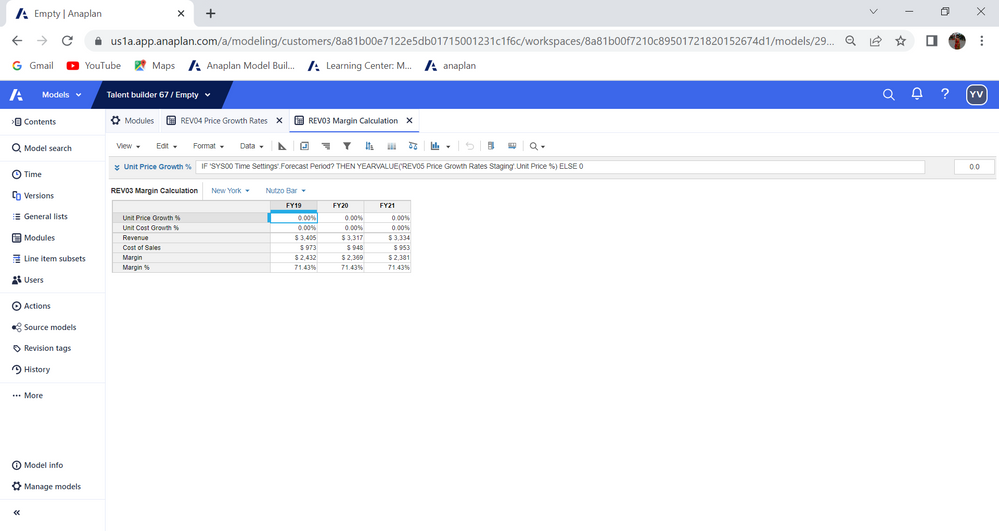tried all of it , not able carryforward it to REV03